SCHOLASTIC APTITUDE TEST 1999

PHYSICS

 Time: ONE Hour Max. Marks: 60

• Answers must be written either in English or the medium of instruction of the candidate in high school.
• Answer all the questions in the booklets provided for the purpose.
• There will be no negative marking.
• The relevant working in arriving at an answer has to be shown wherever required. However if the answer can be arrived at without detailed working, write only the answer
• Use of calculators or graph papers is not permitted.
• All questions carry equal marks

1. At a certain moment of time a particle moving along a straight line is located at a distance of 5 m from a point and at a subsequent moment it is at a distance of 10 m from the same point. The minimum and maximum distances the particle could have moved are                                  .
2. A particle is going round a circular path with a constant speed. The angle between its acceleration and velocity vectors is                                   .
3. An object placed on an equal arm balance requires 12 kg to balance it. When placed on a spring scale, the scale reads 120 N. Everything (balance, scale, set of masses, and the object) is now transported to the moon where the gravitational force is one-sixth that on Earth. The new readings of the balance and the spring scale are                                     respectively.
4. A body is thrown up from a certain height and another thrown down from the same point with the same speed at the same time. If they hit the ground with a time gap of 4 s, the speed of projection is                             .
5. On applying brakes a car slows down from v1 to v2 as it travels a certain distance and from v2 to v3 as it travels an equal distance. the relation between the velocities is                                    .
6. A ball of mass m is thrown vertically upward. Air resistance is not negligible. Assume the force of air resistance has magnitude proportional to the velocity, and direction opposite to the velocity's. At the highest point, the ball's acceleration is                                        (greater than /less than /equal to) acceleration due to gravity.
7. A converging lens with focal length +10 cm would produce a twice magnified image of an object, if the object is placed at a distance of                       from it.
8. Two small identical conducting spheres are separated by a distance much larger than their diameter. They are initially given charges of -2.00 m C and +4.00 m C, and are found to exert a force on each other of magnitude 1 N. Without changing their position, they are connected by a conducting wire. When the wire is removed, the magnitude of the force between them is                              .
9. Three 60 W light bulbs are mistakenly wired in series and connected to a 120 V power supply. Assume the light bulbs are rated for single connection to 120 V. With the mistaken connection, the power dissipated by each bulb is                           .
 In the circuit shown the resistance R1, R2, R3, R4 and R5 are 80 W , 20 W 60 W 25 W and 20 W respectively. A current of 0.10 A flows through the 25 W resistor shown in the diagram. The current through the 80 W resistor is                   .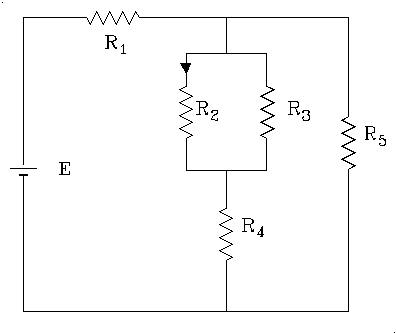1. A wire of a certain radius has a resistance R. Another wire of same length, same material has one end of same radius and the other end of twice greater radius, the radius increasing linearly from the narrower end. If the resistance R' of the second wire is less than nR where n is an integer, n would be                                .
2. Angular momentum of a particle about a point is defined as the product of its mass, velocity and perpendicular distance from the given point. A particle of mass m is moving in the xy plane parallel to x- axis at a distance d from it with a speed v. Its angular momentum about the origin would be
3. An alloy consists of a metal A of density 3.1 gm/cc and a metal B of density 8.8 gm/cc mixed in the ratio 2:1. A hollow ball made of this material has an external volume of 80 cc and a mass of 100 g. The volume of the cavity is                          .
4. A body is dropped from a height of 20 m above the ground. If gravity disappears 1 second after it starts falling , the time it takes to hit the ground is ( Assume acceleration due to gravity = 10 ms–2 )                        .
 The diagram to the right shows the velocity-time graph for two masses A and B that collided elastically. Consider the following statements. I. A and B moved in the same direction after the collision. II. The velocities of A and B were equal at the mid-time of the collision III. The mass of A was greater than the mass of B. The true statements from these are                                           .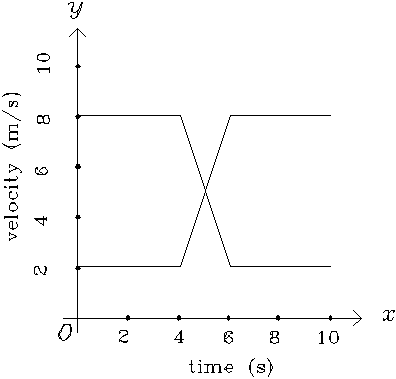Potential difference between A and B is                        and the current flowing from C to D is                     .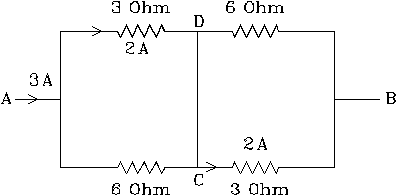1. Parallel beam of light parallel to the principal axis of a lens falls on a lens of focal length 50 cm. After passing through the first lens they pass through another coaxially mounted convex lens of focal length 50 cm placed 150 cm from the first lens. After passing through the second lens, the rays converge at a distance of __________ from the first lens.
2. If velocity of light in medium A is 2.25 ´ 108 m/s. Refractive index of medium B with respect to medium C is 1.2. Velocity of light in a medium C is 1.5 ´  108 m/s. Refractive index of A with respect to B is                                 . ( You should not use the value of velocity of light in vaccum or air ).
3. Particles A and B are free to move along parallel paths. B is moving at a constant velocity of 10 m/s and A starts moving at the moment B passes it. If A accelerates at 1 m/s2 for 5 s and then travels at a constant velocity, the distance between A and B 10 s after B passes A is                     .
4. Dust particles are uniformly distributed in space with a mass per unit volume r . A circular plate of area A is moving in this space at v. The dust particles that come in to contact with the plate stick to it. The mass of dust that collects on the plate in a time t is                                  .
 15 equal resistances (AB,BC,CD,…….) are connected to form a star as shown. When the resistances JB and JH are removed the equivalent resistance between terminals A and I is R. The equivalent resistance between A and I when the resistances AJ and JI are removed would be                     .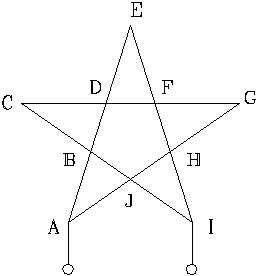1. A point object is located 5 cm above the principal axis of a lens of focal length 20 cm. If the distance of the object measured parallel to the principal axis is 30 cm, the distance of the image of the object from the principal axis would be                                     .
2. The orbital radius of a certain satellite is R and it takes two hours to complete one revolution. The radius of the orbit of a satellite that would take 16 hours to complete one revolution would be                                        .
3. A longitudinal wave is normally incident on a wall and is reflected. The incident and the reflected waves interfere producing a stationary wave. If the nearest node to the wall is 25 cm from it and the velocity of longitudinal waves in air is 350 m/s, the frequency of the wave is                            .
4. An solid object A has a mass m and is made of a material of density r . One fourth of its mass is removed and another material of density r /2 is added to it and a new solid object B is formed. The mass of B is 5/4 times the mass of A. The difference in the volumes of the two spheres is                                        .
5. A sealed evacuated vessel of volume 1500 m3 and mass 3 ´ 105 kg is floating on water. The downward force that should be exerted on the vessel to just submerge it is                               .( Density of water is 1 gm/cc, assume g =10 m/s2)
6. A wagon of mass M is free to roll on frictionless rails and is located on a straight track. A man of mass m is standing at one end of the wagon. The man runs parallel to the rails towards the other end and jumps off. If the speed of the man at the moment he jumps off is v, the speed of the wagon is                             .
7. Gravity at a height h above the earth is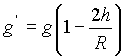for h<<R and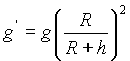for any h.
and gravity at a depth d below the earth is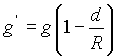for all d.
( R= 6400 km, g is the gravity on the earth and g' gravity at the specified position)
Use these equations in filling the following blanks.

The height where the gravity is same as at a depth of 10 km is
The height where the gravity is same as at a depth of 3200 km is                             .
8. The fundamental frequency of vibration of a string stretched between two supports is given by the expression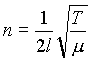, where n, l, T and m are the frequency, length of the string, tension in the string and mass per unit length respectively. Use this equation in filling the following blank.

A string stretched between two supports has a frequency n. This string is then stretched to twice its original length and fixed between two new supports with same tension as earlier. The new fundamental frequency is                                      .

1. A charged particle moving northward at right angles to a magnetic field experiences a force directed eastward. Another charged particle of opposite charge is moving towards southeast in the same magnetic field. The force experienced by it is directed towards                                  .